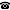# Introduction to the Einstein equations as an evolution problem

Talk held by Jérémie Joudioux (Radboud University Nijmegen, Netherlands) at the KGRC Friday seminar on 2018‑05‑04.

### Abstract

The theory of relativity is a cornerstone of the contemporary physics. As its center lies the Einstein equations which couple the curvature of a space-time to the distribution of matter. As a mathematician, these equations are the source of numerous difficult physically relevant problems mixing analysis and differential geometry. The purpose of the talk is to introduce the Einstein equations as an evolution problem, to understand the meaning of the initial value problem for the associated pde. If time allows, we will have a glimpse at contemporary problems in relativity. The talk is an introduction, we hope the audience will be able to make several leaps of faith.

Kurt Gödel Research Center for Mathematical Logic. Währinger Straße 25, 1090 Wien, Austria.+43-1-4277-50501. Last updated: 2018-05-08, 16:01.